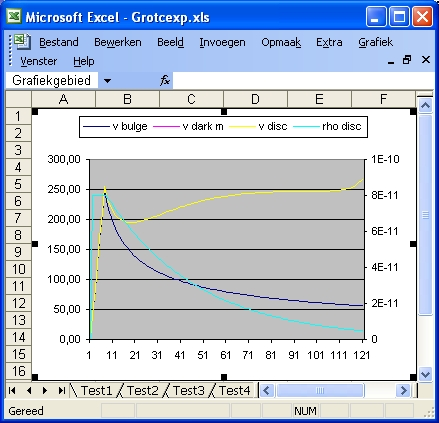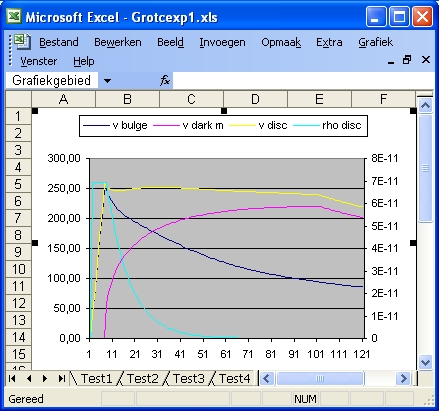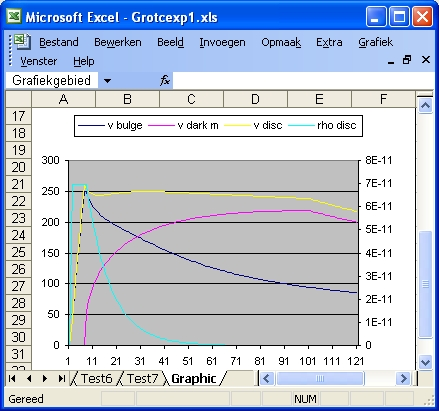## Description of Excel program: Grotcexp.xls and Grotcexp1.xls

The purpose of the Excel programs is to calculate your own galaxy rotation curve as a function of a bulge, a disk and a halo of darkmatter. This halo is an option
This procedure goes in two steps:
• First you enter the parameters with the dark matter parameters equal to zero, and then you observe the galaxy rotation curve. This rotation represents the expected curve based on visible mass. The strategy should be that the rotation curve matches the visible mass.
• Secondly you change the dark matter parameters and you observe the galaxy rotation curve. The strategy should be that the rotation curve becomes flat. This rotation curve represents the observed curve. If not you try again.
There are two dark matter profiles considered: NFW and Hernquist
For a copy of the program in zip format select grotc.xls.zip. This file also contains the programs grotc.xls and grot1.xls. For a discription of both See: grotc.xls
The main physical assumption is that visible matter and darkmatter behave similar. In this simulation for both visible matter and dark matter this is Newton's Law
The main problem or chalenge is not to include to much darkmatter, at least such that the darkmatter density should not be higher than the visible matter, because otherwise it will become visible.

In Excel the distance of the galaxy rotation curve is expressed in Units. One unit is identical with one line of information (results) and is equivalent with 1000 Lightyears.
The average radius of a rotation curve is 100 units or 100000 Lightyears or 30 kpc.
The standard distance of the bulge is 6 units or 6000 Lightyears. This distance is defined by the parameter rbulge. Within this range the speed of the rotation curve increases lineair to 250 km/sec. There after the speed is calculated as a function of the density.
The program consists of approximate 6 tests: Test1, Test2, Test3, Test4, Test5 and Test6
Each test has 11 parameters: "NFW", "r bulge", "r disk", "rdisp", "rs" , "rhos", "a", "Mh", "rho disk str", "rho disk end" and "Rd"

• The variable "NFW" (or "Hernquist") is used to select the NFW profile. When NFW is 1 the NFW profile is selected. When NFW is 0 the Hernquist profile is selected. When this is the case the description changes into "Hernquist" and the value into 1. When Hernquist is 0 the reverse happens.
• The variable "r bulge" shows the length of the bulge in Lightyears. The maximum value is 100000.
• The variable "r disk" shows the length of the disk in Lightyears. The maximum value is 400000.
• The variable "r disp" shows the length of the disk in Lightyears. The maximum value is 400000.
• "rs" and "rhos" are two parameters of the NFW dark mattter profile. The dimension of rs is in Lightyears. See http://lanl.arXiv.org/pdf/astro-ph/0301144 page 17 for more details.
• "a" and "Mh" are two parameters of the Hernquist dark mattter profile. The dimension of "a" is in Lightyears. The dimension of Mh is in 10^12*m0, with m0 being the mass of the Sun. See http://lanl.arXiv.org/pdf/astro-ph/0506015 page 2 for more details.
• "rho disk s", and "Rd" are input parameters to calculate the disk density profile. The dimension of Rd is in Lightyears. The parameter "rho disk end" is calculated at the distance of "rdisk". See below

In order to start the simulation select START button.

Each simulation consists only of 1 phase. In effect this phase is the same as phase 4 of the Excel programs Circ11.xls, Circ12.xls etc.

In this phase the galaxy rotation curve is calculated as a function of the density of the bulge, the disk and the density of dark matter profile (either NFW or Hernquist).
In the programs Circ11, Circ12 etc the density profile of the disk was calculated based starting from a flat rotation curve (if VSEL = 1). For a description of that program select circ11.xls.htm
In this program grotcexp.xls the density is calculated in two ways:

• When r <= rbulge then rho = (v/rbulge)^2 * (3*G/4*pi) with v(rbulge) = 250 km/sec
In this range rho is constant because v(r)/r is also constant.
When rhodisks = 0 then rhodisks = rho.
• when r > rbulge with the following formula :
rho = rhodisks * exp (- r/Rd)
For a different disk shape See: grotc.xls.

For readers not familiar with rotation curves select this overview article by Stephen M. Kent: http://adsbit.harvard.edu/cgi-bin/nph-iarticle_query?bibcode=1987AJ.....93..816K
There is also a PDF version available from that link. Rememember that Andromeda galaxy, M31 and N224 are one and the same.

### Description of Excel program: Grotcexp.xls - No Dark matter

#### Test1, Test3 and Test5

The purpose of Test1 is to calaculate the Galaxy Rotation Curve for a certain value of Rd.
It is important in order to do this test to set both the parameters rho disk start and rho disk end to zero and select "Start". The reason to set the start value of the disk density equal to the density of the bulge.
Observe Column "D" and "K" and establish when the density is roughly 1E-11. Also study the speed in the range of the disk. This is the yellow line.
• In Test1, with Rd = 30000 the maximum speed is roughly 210 km/sec. The 1E-11 rho disk density value is reached at r = 65000 Ly
• In Test3, with Rd = 40000 the maximum speed is roughly 240. The 1E-11 value is reached at r = 84000 Ly
• In Test5, with Rd = 10000 the speed slows down continuous. The 1E-11 vlalue is reached at r = 26000 Ly

#### Test1 and Test2

The purpose of Test1 versus Test2 is to observe what happens if you increase the parameter rho disk start. The parameter Rdis the same in both.
Observe Column "D" rho bulge and "K" rho disk.
• In Test1, with rho disk start = 7E-11 the maximum speed is roughly 210 km/sec. The 1E-11 rho disk density value is reached at r = 65000 Ly
• In Test2, with rho disk start = 1.05E-10 the maximum speed is roughly 250. The 1E-11 value is reached at r = 77000 Ly

#### Test3 and Test4

The purpose of Test3 versus Test4 is to observe what happens if you increase the parameter rho disk start. The parameter Rdis the same in both.
Observe Column "D" rho bulge and "K" rho disk.
• In Test3, with rho disk start = 7E-11 the maximum speed is roughly 210 km/sec. The 1E-11 rho disk density value is reached at r = 84000 Ly
• In Test4, with rho disk start = 8E-11 the maximum speed is roughly 250. The 1E-11 value is reached at r = 90000 LyTest 4

#### Test4, Test6 and Test7

The purpose of these 3 test is to observe what happens if you increase the parameter "r disk". The parameter Rd is the same and equal to 40000. The result is that the mass of the disk will increase.
• The 3 values of "r disk" are: 120000, 300000 and 400000 Ly.
• The total (bulge + disk) mass values are: 69.4E40, 84.9E40 and 85.3E40
• The smallest disk density values are: 4.74E-12, 5.27E-14 and 4.33E-15
• The density level of the bulge is 6.94E-11. The density level at a distance of 197000 Ly is 6.92E-13. This is a factor 100 lower.
The importance of these 3 test is that in order to do a proper simulation you should continue at least until r = 200000 Ly.

### Parameters of Excel program Grotcexp.xls

 Parameter Test1 Test2 Test3 Test4 Test5 Test6 Test7 1 NFW 1 1 1 1 1 1 1 2 rbulge 6000 6000 6000 6000 6000 6000 6000 3 disk 110000 110000 120000 120000 100000 300000 400000 4 NFW rs 0 0 0 0 0 0 0 5 NFW rhos 0 0 0 0 0 0 0 6 Hq a 0 0 0 0 0 0 0 7 Hq MHalo 0 0 0 0 0 0 0 8 Rho disk s 6,94E-11 10,5-11 6,94E-11 8E-11 6,94E-11 8E-11 8E-11 9 Rho disk e 2,24E-12 3,38E-12 4,11E-12 4,74E-12 6,34E-13 5,27E-14 4,32E-15 10 Rd 30000 30000 40000 40000 40000 40000 40000 11 Rho NFW 0 0 0 0 0 0 0 12 tot m bulge 5,32 5,32 5,32 5,32 5,32 5,32 5,32 13 tot m disk 36,3 54,89 55,63 64,10 6,87 79,67 80,01 14 tot m dm 0 0 0 0 0 0 0 15 max r dm 0 0 0 0 0 0 0

### Description of Excel program: Grotcexp1.xls

#### Test1, Test2, Test3 and Test4

The purpose of the four tests is to simulate the observed flat rotation curve by introducing dark matter and by using the NFW profile.
The amount of visible mass in all simulations is the same i.e the density function parameters "rho disk start", and "Rd" RD are the same.
• The parameter rs is selected first for each of the 4 tests. This paramater defines the peak of the profile. The 4 values are: 7500, 15000, 30000 and 48000 Ly. The 4 peak values are at 15000,30000, 60000 and 96000 Ly,
• Next the parameter "rhos" (density) is selected. The 4 values are selected that the maximum speed is approximate equal to the maximum speed at the rim of the bulge. The 4 values are: 40E-12, 10E-12, 3.5E-12 and 1.35E-12.
• The result of Test4 is almost a complete flat galaxy rotation curve.Test 4

#### Test5, Test6 and Test7

The purpose of the three tests is to simulate the observed flat rotation curve by introducing dark matter and by using the Hernquist profile.
The density function parameter RD is the same as used in the four tests above.
• The parameter a is selected first for each of the 3 tests. This paramater defines the peak of the profile. The 3 values are at 30000, 60000 and 96000 Ly,
• Next the parameter "Mh" (Mass Halo) is selected. The dimension of Mh is in 10^12*m0, with m0 being the mass of the Sun. The 3 values are selected that the maximum speed is approximate equal to the maximum speed at the rim of the bulge. The 3 values are: 0.4 0.8 and 1.35 (Times 2*10^42 Kg)
• The result of Test7 is almost a complete flat galaxy rotation curve.
This result is identical as Test4Test 7

### Parameters of Excel program Grotcexp.xls

 Parameter Test1 Test2 Test3 Test4 Test5 Test6 Test7 1 NFW 1 1 1 1 0 0 0 2 rbulge 6000 6000 6000 6000 6000 6000 6000 3 disk 100000 100000 100000 100000 100000 100000 100000 4 NFW rs 7500 15000 30000 48000 0 0 0 5 NFW rhos 4E-11 1E-11 3.2E-12 1,35E-12 0 0 0 6 Hq a 0 0 0 0 30000 60000 96000 7 Hq MHalo 0 0 0 0 0,4 0,85 1,35 8 Rho disk s 6,94E-11 6,94E-11 6,94E-11 6,94E-11 6,94E-11 6,94E-11 6,94E-11 9 Rho disk e 3,12E-12 6,63E-13 6,34E-15 6,34E-15 6,34E-15 6,34E-15 6,34E-15 10 Rd 10000 10000 10000 10000 10000 10000 10000 11 Rho NFW 1E-11 2.5E-12 8E-13 3,375E-13 0 0 0 12 tot m bulge 5,32 5,32 5,32 5,32 5,32 5,32 5,32 13 tot m disk 6,87 6,87 6,87 6,87 6,87 6,87 6,87 14 tot m dm 159 322 853 1490 75 164 264 15 max r dm 17951K 359021K 919112K 1588234K 6883K 14193K 22624K

#### Discussion

What those tests tell you is
• That it is very easy to simulate the flat galaxy rotation curves by using either the Hernquist or the NFW profile.

What those tests don't tell you is

• Which of the two profiles is correct.
• If the concept of darkmatter (nonbaryonic) is the true solution of the missing mass problem.

### Literature

Created: 23 January 2007